# 2 相关技术

## 2.1 对数变换法图像增强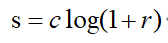gs= im2uint8(mat2gray(g));


## 2.2 指数变换法图像增强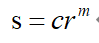## 2.3 图像灰度直方图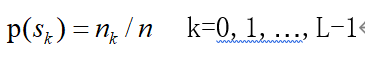for i=1:256         %查找ima矩阵中各个值的个数，并且放入数组中
x(i)=size(find(ima==(i-1)),1);  %find函数对ima查找，统计查找的个数
y(i)=x(i)/(m*n);        %各个元素的概率
end


## 2.4 直方图均衡化增强

1、像素无论怎样映射，一定要保证原来的大小关系不变，原来较亮的区域依然较亮，较暗的区域依然较暗，只是和以前相比，对比度拉升，灰度值大小关系没有改变；
2、像素映射的值域应该在0L-1，不能越界，实验中灰度级是八位，所以像素映射函数的值域是0255之间。

1、统计直方图每个灰度级出现的次数。用一个一维矩阵来存放0~255每个灰度级出现的次数，并且计算出每个像素出现的概率（归一化）。
2、累计归一化的直方图。计算出每个灰度值对应的累计分布。
3、计算新的像素值。累计分布函数乘以（L-1）得到新的像素值。
4、将原图像灰度值映射到新的像素值中。

# 3 实验结果与分析

## 3.1 对数变化法图像增强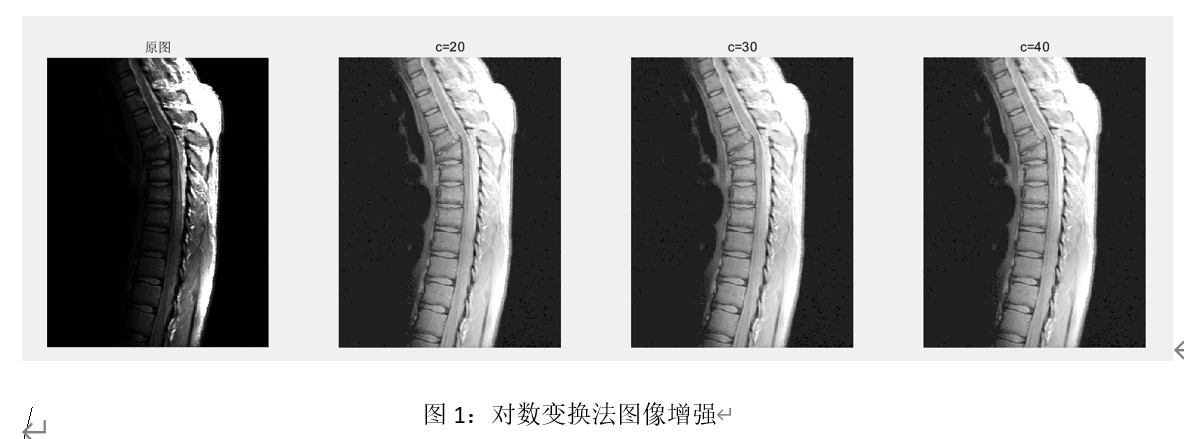## 3.2 指数变换法图像增强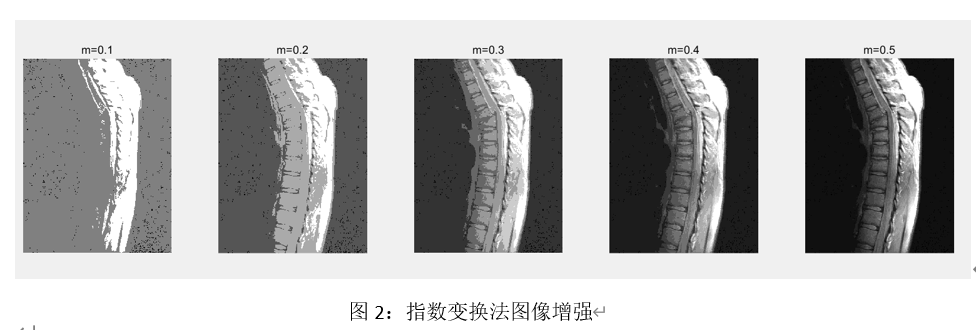## 3.3 直方图均衡化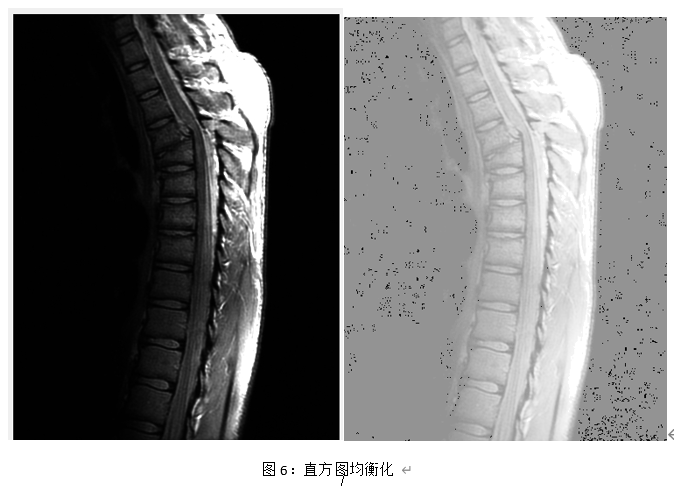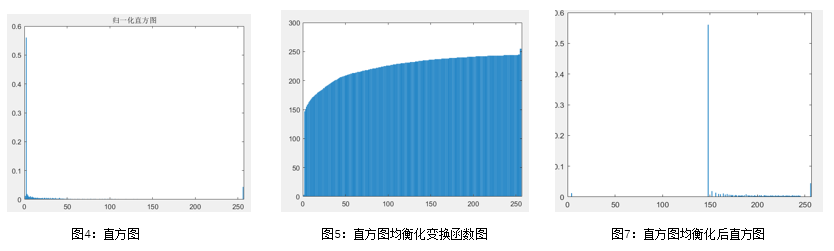# 4 程序

## 4.1 对数变换方法进行图像增强

ima = imread('C:\Users\machenike\Desktop\DIP\pro3\Fig3.08(a).jpg');
s20=uint8(20*(log(1+double(ima))));
s20=im2uint8(mat2gray(s20));
figure,
subplot(1,4,1),  imshow(ima);title('原图');
subplot(1,4,2),  imshow(s20);title('c=20');

## 4.2 指数变换方法进行图像增强
s1=uint8(double(ima).^(0.1));%   整数只能升为整数幂，需要对ima取double型，imshow可以显示浮点型和整形
s1=im2uint8(mat2gray(s1));
subplot(1,5,1),  imshow(s1);title('m=0.1');


## 4.3 计算图像直方图

function [x,y]=pro3_3(ima)
[m,n]=size(ima);    %计算图像的行列数
for i=1:256         %查找ima矩阵中各个值的个数，并且放入数组中
x(i)=size(find(ima==(i-1)),1);  %find函数对ima查找，统计查找的个数
y(i)=x(i)/(m*n);        %各个元素的概率
end
figure,bar(y);title('归一化直方图');
End


## 4.4 直方图均衡化

ima0 = imread('C:\Users\machenike\Desktop\DIP\pro3\Fig3.08(a).jpg');
figure,imshow(ima0);  %显示原图像
pro3_3(ima0);             %绘制直方图
ima=ima0+1;   %全部加1，矩阵下标必须为正整数或者逻辑数据
[m,n]=size(ima0);    %计算图像的行列数
for i=1:256         %查找ima矩阵中各个值的个数，并且放入数组中
x(i)=size(find(ima==i),1);  %find函数对ima查找，统计查找的个数
y(i)=x(i)/(m*n);        %各个元素的概率
end

sum=zeros(256,1);  %存放分布概率
z=zeros(256,1); %存放映射的灰度值

%求累积分布和映射的灰度值
for i=1:256
if i==1
sum(1,1)=y(1);
else
sum(i,1)=y(i)+sum(i-1);
end
z(i)=uint8(sum(i)*256);
end
ima1=zeros(m,n);
figure,bar(z);
%对灰度值进行映射
for j=1:m
for k=1:n
ima1(j,k)=(z(ima(j,k)));
end
end
ima1=uint8(ima1);  %对均衡化后的图像取整
figure,imshow(ima1);
pro3_3(ima1);


10-301727

#### 数字图像处理习题（直方图均衡化）

08-122869

#### 图像增强算法（直方图均衡化、拉普拉斯、Log、伽马变换）04-191540

#### app随着光照强度变化总结

01-145729

#### 【图像处理】图像强度变换、直方图均衡化（Image Intensity Transformations and Histogram Equalization）

06-011220

#### 【图像处理】 matlab 实现彩色图像的直方图均衡化算法

11-083491

#### 图像强度归一化Intensity normalization

03-06850

#### CVPR最新医学影像AI论文：利用学习图像变换进行数据增强

05-082万+

#### 图像处理总结概念

08-123万+

#### 图像分割 和 边缘检测

12-184449

#### 关于图像对比度【4】——对比度调整强度©️2020 CSDN 皮肤主题: 编程工作室 设计师: CSDN官方博客点击重新获取扫码支付1.余额是钱包充值的虚拟货币，按照1:1的比例进行支付金额的抵扣。
2.余额无法直接购买下载，可以购买VIP、C币套餐、付费专栏及课程。余额充值